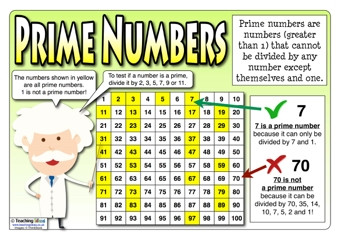## Generate Prime Numbers in VB6 2022

Home / Generate Prime Numbers in VB6 2022Source: http://www.teachingideas.co.uk/multiplication/prime-numbers

Prime numbers are the numbers that are greater than 1 and divisible by one and by itself, while the composite numbers are not. Some examples are: 2,3,5,7,11
you''ll notice that majority of the prime are odd numbers and the number 2 is the only even number that is prime because 2 is divisible by one and by itself (2/2)

let''s create a prime number generator in VB6, I don''t know if my algorithm is efficient, it outputs the prime numbers correctly but it is sluggish when it comes to program execution.

''Here is the code

''The program will output the prime numbers (via message box) according to the number of input

``````Function output_prime(number as long)

Dim counter as long

counter = 0

''use for loop

For A=1 to number

For B=1 to number

If (A Mod B = 0) Then

counter = counter + 1

End If

Next B

''prime is divisible only by two numbers, if the counter is >2 then the current number is composite

If (counter == 2) Then

''You can change method of showing the output (listbox,combobox), I just use the message box as an example
msgbox A

End If

counter = 0

Next A

End Function

''Call the function
call output_prime(1000)``````

The program uses brute force to check the number, e.g if the input is 1000; the program will divide each number from 1 to 1000, thus the program execution is slow when you input large number.